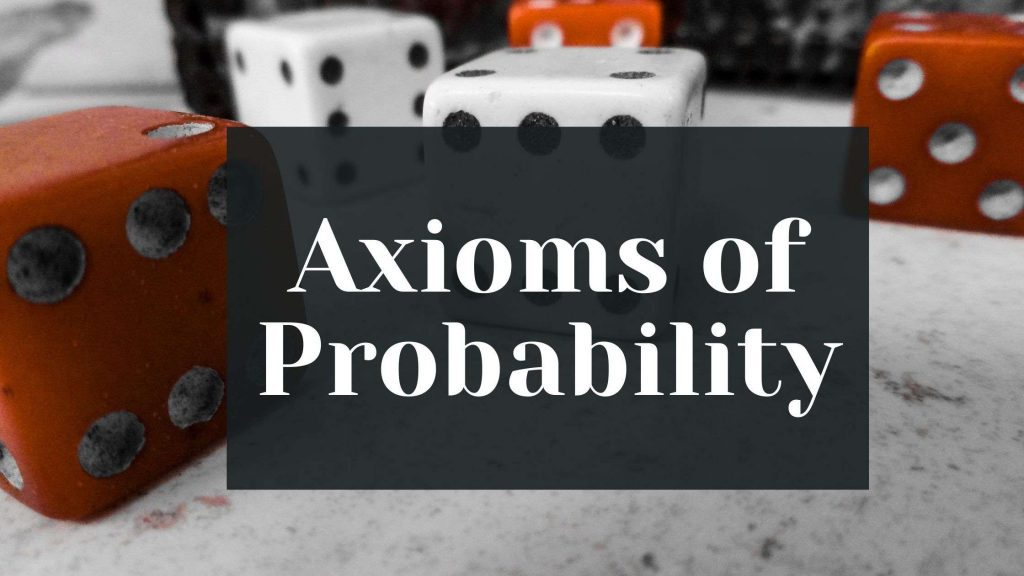Maths
Study Material

# Axioms of Probability and Solved Problems

This blog contains the rules of probability, which are a must to know to solve problems of probability. It also has solved problems on the topics discussed.

3 minutes long
Posted by Tanwir Silar, 25/8/2021Hesap Oluştur

Got stuck on homework? Get your step-by-step solutions from real tutors in minutes! 24/7. Unlimited.

The classical theory of probability can not be used when the outcomes of a experiment are not equally likely. On the other hand, Axiomatic approach to probability can be used whether or not the outcomes of an experiment are equally likely. The axioms of probability are explained in this blog.

## Axioms of Probability

Let S be the sample space of a random experiment. The probability P is a real valued function whose domain is the power set of S and range is the interval [0,1].
There are three axioms of probability. Good understanding of these axioms is necessary to crack any problem of probability.

Axiom 1:
For any event E, P(E) ≥ 0.
This main conclusion of this axiom is that the probability of an event is a non – negative number.

Axiom 2:
P(S) = 1, i.e., the probability of occurrence of sample space is 1.

Axiom 3:
If E and F are mutually exclusive events, then P(E ∪ F) = P(E) + P(F).
It follows from axiom 3 that P(Φ) = 0, where Φ is null set.

## Solved problems

Look at the following solved problems for better understanding of the concept.

### Example 1:

Question: There are 3 pieces taken from a lot. What is the probability that exactly two of them are defective?

Solution: Assigning the letter B for defective and G for non-defective/good pieces, let us firstly list out all the possible outcomes, i.e., the sample space.
There are outcomes with
(i) no defective pieces {GGG}.
(ii) exactly one defective piece {BGG, GBG, GGB}
(iii) exactly two defective pieces {BBG, BGB, GBB}
(iv) all defective pieces {BBB}.

Our sample space S = {GGG, BGG, GBG, GGB, BBG, BGB, GBB, BBB}
The event that exactly two of the pieces are defective E = {BBG, BGB, GBB}
Here, since the outcomes are equally likely. P(E) = n(E)/ n(S)
n(E) = 3, n(S) = 8. P(E) = 3/8.

### Example 2:

Question: One card is drawn from a well shuffled deck of 52 cards. If each outcome is equally likely, calculate the probability that the card will be
(i) a diamond (ii) not a diamond (iii) not an ace

Solution:
Since, it has been given that all the outcomes are equally likely, we can use the formula P(E) = n(E)/n(S).
As a single card is drawn, the sample space consists of each and every card of the deck as a sample point.
n(S) = 52.
(i) probability that card is a diamond is
E be the event that the card drawn is a diamond. E = { card : card is a diamond}
n(E) = number of diamonds in a deck = 13.
P(E) = n(E)/n(S) = 13/52 = 1/4.

(ii) probability that the card is not a diamond is
If E is the event that the card drawn is a diamond, then E’ (complement of E) is the event we are looking for in this sub problem.
We know that P(E ∪ E’) = P(E) + P(E’) = P(S) (from set theory) and P(S) = 1.
As we calculated P(E) in the previous sub problem,hence it should be easy to calculate P(E’).
So, P(E’) = 1 – P(E) = 1 – 3/8 = 5/8.

(iii) probability that card is not an ace is
Let E be an event that the card is an ace. n(E) = number of ace cards in a standard deck = 4.
Then the answer is P(E’) (E’ is the event that the card is not an ace)
P(E) = n(E)/n(S) = 4/52 = 1/13.
P(E’) = 1 – P(E) = 1 – 1/13 = 12/13.